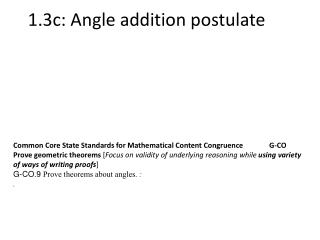Download Presentation1.3c: Angle addition postulate - PowerPoint PPT Presentation

1.3c: Angle addition postulate. Common Core State Standards for Mathematical Content Congruence G-CO Prove geometric theorems [ Focus on validity of underlying reasoning while using variety of ways of writing proofs ] G-CO.9 Prove theorems about angles. :. Angle Addition Postulate.I am the owner, or an agent authorized to act on behalf of the owner, of the copyrighted work described.
Download PresentationDownload Policy: Content on the Website is provided to you AS IS for your information and personal use and may not be sold / licensed / shared on other websites without getting consent from its author.While downloading, if for some reason you are not able to download a presentation, the publisher may have deleted the file from their server.

- - - - - - - - - - - - - - - - - - - - - - - - - - E N D - - - - - - - - - - - - - - - - - - - - - - - - - -
Presentation Transcript
1. 1.3c: Angle addition postulate Common Core State Standards for Mathematical Content Congruence G-CO Prove geometric theorems [Focus on validity of underlying reasoning while using variety of ways of writing proofs] • G-CO.9 Prove theorems about angles. : .

2. Angle Addition Postulate • If R is in the interior of PQS, then m PQR + m RQS = m PQS. P R 30 Q 20 S

3. Find the m< CAB

4. Example of Angle Addition Postulate 100 Ans: x+40 + 3x-20 = 8x-60 4x + 20 = 8x – 60 80 = 4x 20 = x 40 60 Angle PRQ = 20+40 = 60 Angle QRS = 3(20) -20 = 40 Angle PRS = 8 (20)-60 = 100

5. Find the m< BYZ -2a+48 4a+9 4a+9

6. Angle bisector: a ray that bisects an angle into two congruent angles

7. E H F G

8. 1. Find x.

9. Last example: Solve for x. BD bisects ABC A x+40=3x-20 40=2x-20 60=2x 30=x D x+40o 3x-20o C B Why wouldn’t the Angle Addition Postulate help us solve this initially?

10. In class assignment Homework: Page 50: Evens 18-28, 33-35